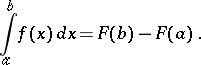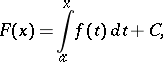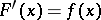# Newton-Leibniz formula

(diff) ← Older revision | Latest revision (diff) | Newer revision → (diff)

The formula expressing the value of a definite integral of a given functionover an interval as the difference of the values at the end points of the interval of any primitive (cf. Integral calculus)of the function:(*)

It is named after I. Newton and G. Leibniz, who both knew the rule expressed by (*), although it was published later.

Ifis Lebesgue integrable overandis defined bywhereis a constant, thenis absolutely continuous,almost-everywhere on(everywhere ifis continuous on) and (*) is valid.

A generalization of the Newton–Leibniz formula is the Stokes formula for orientable manifolds with a boundary.